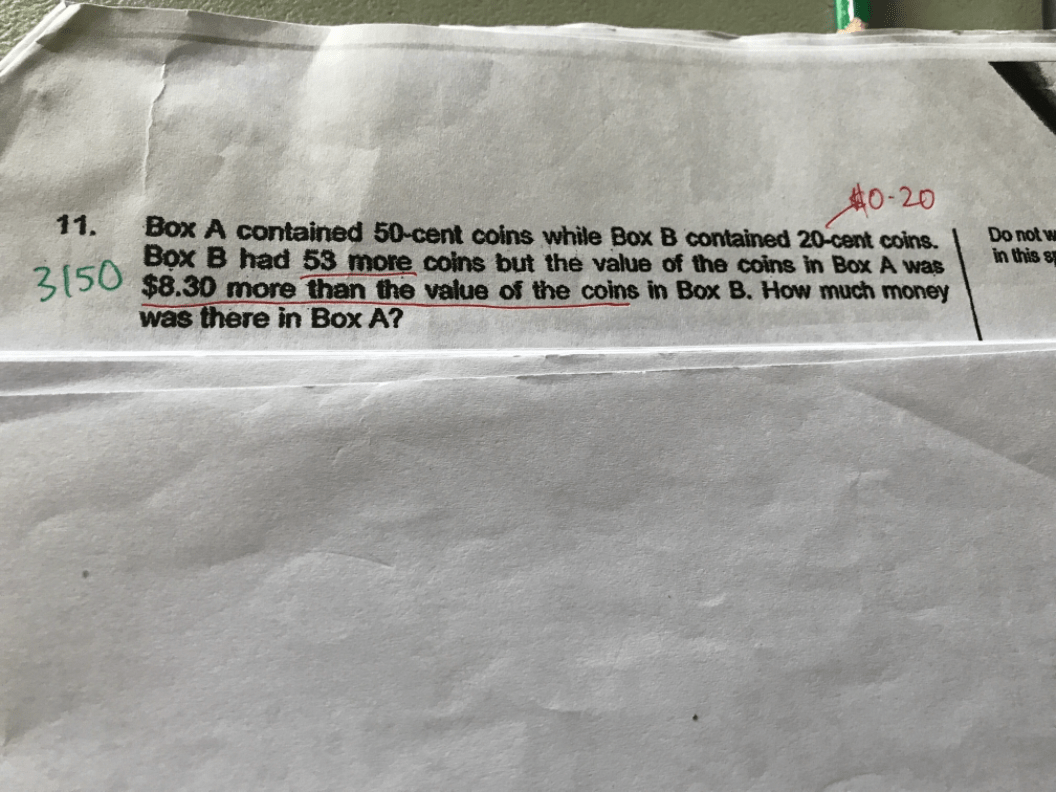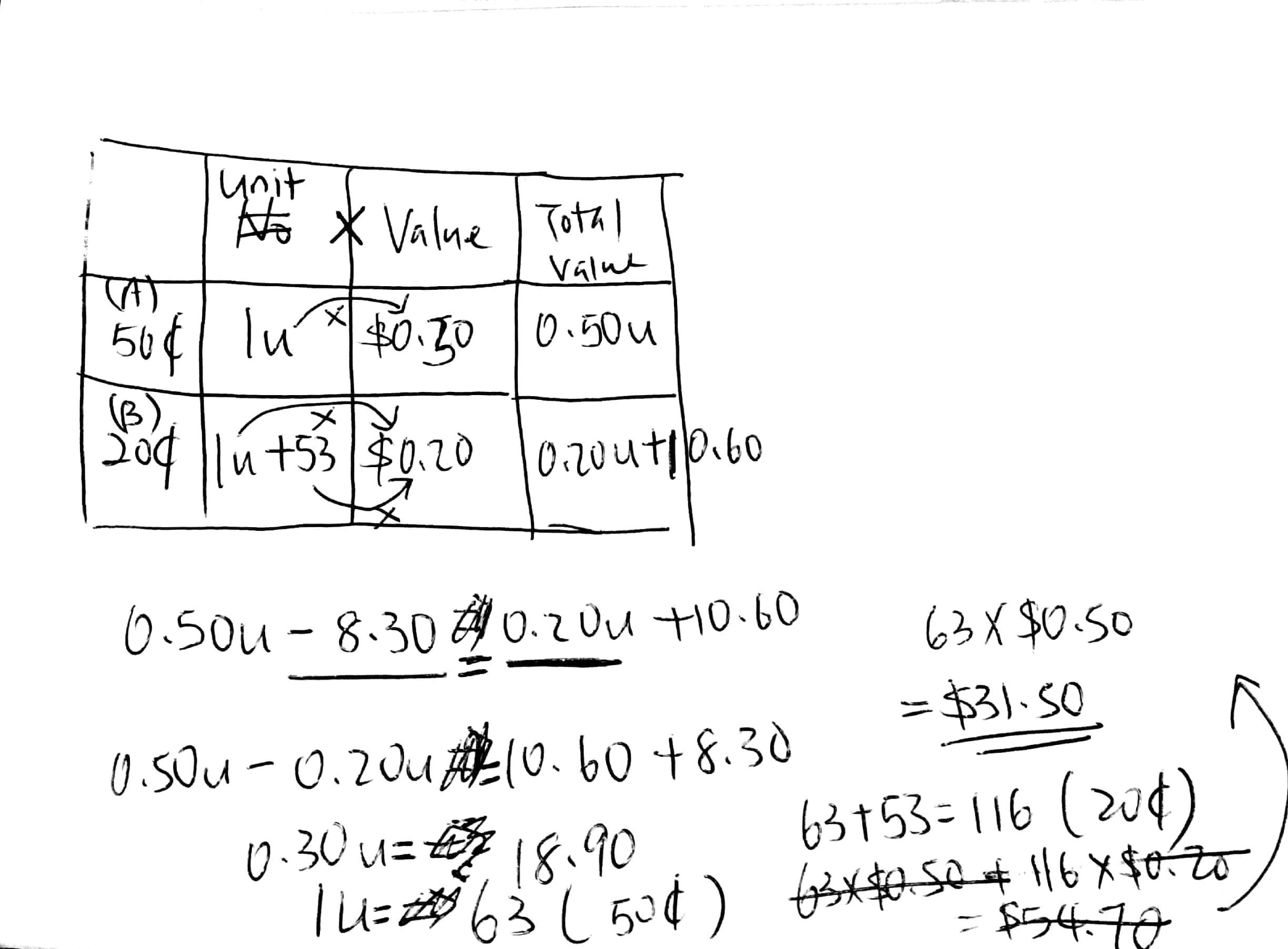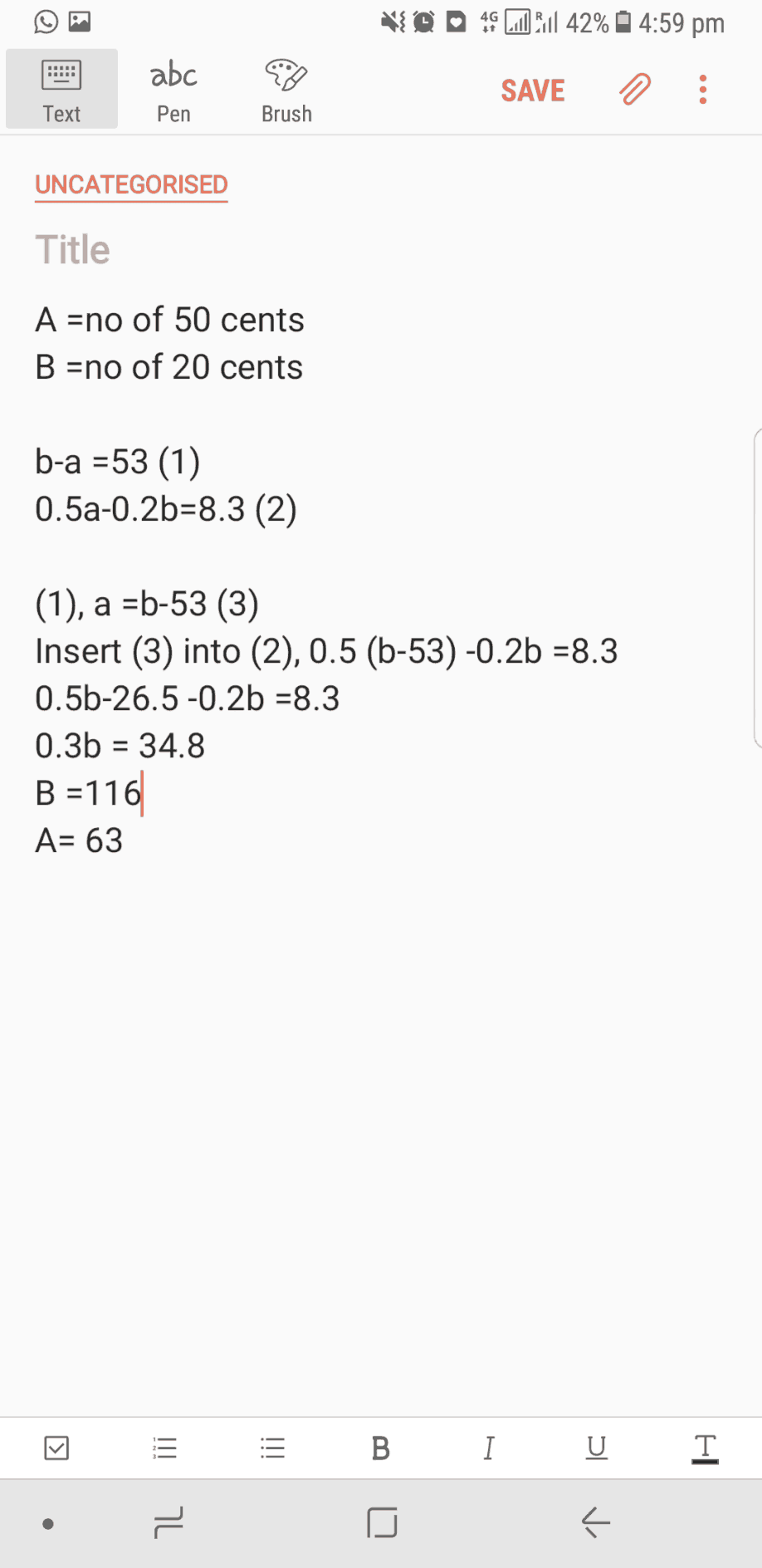# QuestionNeed some guide to solve this question.

is it using comparison or units x value?

Source: Nan Hua Primary

4 Answers

# AnswerThis is using unit x value method. Hope this helps you.

0 Replies 1 Like ✔Accepted Answer

Box A (50-cent coins) : Box B (20-cent coins)
1 unit : (1 unit + 53) [number of coins)

1 unit x 0.50 – (1 unit + 53) x 0.20 = 0.3 unit – 10.60 = 8.30
1 unit = (8.30 + 10.60)/0.30 = 31.50

Ans : \$31.50.

0 Replies 0 Likes1 Reply 0 Likes

Thanks

0 Replies 0 Likes